#### Ergodicity

In addition to the constant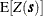and the assumption of intrinsic stationarity, ergodicity is a necessary third hypothesis to estimate the empirical semivariance. Assume that for the SRF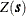you have measurements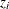whose sample mean is estimated by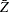. The hypothesis of ergodicity dictates that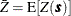.

In general, an SRFis characterized as ergodic if the statistical moments of its realizations coincide with the corresponding ones of the SRF. In spatial analysis you are often interested in the first two statistical moments, and consequently a more relaxed ergodicity assumption is made only for them. See Christakos (1992, section 2.12) for the use of the ergodicity hypothesis in SRF, and Cressie (1993, p. 57) for a more detailed discussion of ergodicity.

The semivariogram analysis makes implicit use of the ergodicity hypothesis. The VARIOGRAM procedure works with the residual centered values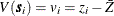,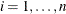, where it is assumed that the sample meanis the constant expected valueof. This is equivalent to using the original values, since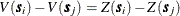, which shows the property of the semivariance to filter out the mean. See the section Semivariance Computation for the exact expressions PROC VARIOGRAM uses to compute the empirical classical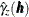and robust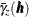semivariances.